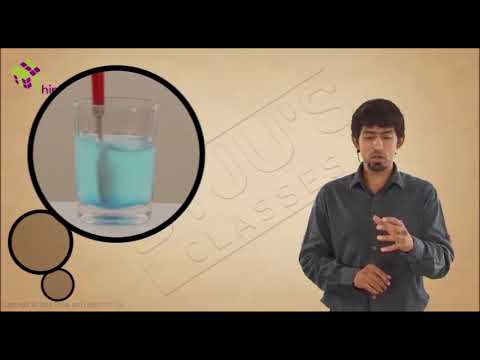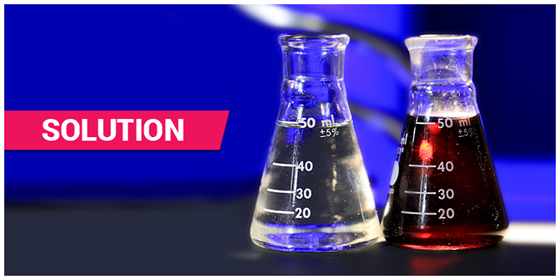# Solution - Properties of Solution

## What is a Solution?

A solution is a homogeneous mixture of two or more components in which the particle size is smaller than 1 nm.

Common examples of solutions are the sugar in water and salt in water solutions, soda water, etc. In a solution, all the components appear as a single phase. There is particle homogeneity i.e. particles are evenly distributed. This is why a whole bottle of soft drink has the same taste throughout.

## Recommended Videos## Characteristics of Solution

Solutions have two components, one is solvent and the other is solute.

### 1. What is a Solvent?

The component that dissolves the other component is called the solvent.

### 2. What is Solute?

The component(s) that is/are dissolved in the solvent is/are called solute(s).

Generally solvent is present in major proportion compared to the solute. The amount of solute is lesser than the solvent. The solute and solvent can be in any state of matter i.e. solid, liquid and gas.

Solutions that are in the liquid state consist of a solid, liquid or gas dissolved in a liquid solvent. Alloys and air are examples of solid and gaseous solutions, respectively.

### 3. Solution Examples

The following examples illustrate solvent and solute in some solutions.

• Air is a homogeneous mixture of gases. Here both the solvent and the solute are gases.
• Sugar syrup is a solution where sugar is dissolved in water using heat. Here, water is the solvent and sugar is the solute.
• Tincture of iodine, a mixture of iodine in alcohol. Iodine is the solute whereas alcohol is the solvent.Solution in Chemistry

## Types of Solution

Liquid solutions, such as sugar in water, are the most common kind, but there are also solutions that are gases or solids. Any state of matter (solid, liquid, or gas) can act both as a solute or as a solvent during the formation of a solution. Therefore, depending upon the physical states of solute and solvent, we can classify solutions in nine different types.

 S.No Types of Solution Solute Solvent Examples 1 Solid-solid solid solid Alloys like brass, bronze etc. 2 Solid-liquid solid liquid The solution of sugar, salt etc in water. 3 Solid-gas solid gas Sublimation of substances like iodine, camphor etc into the air. 4 Liquid-solid liquid solid Hydrated salts, mercury in amalgamated zinc, etc. 5 Liquid-liquid liquid liquid Alcohol in water, benzene in toluene 6 Liquid-gas liquid gas Aerosol, water vapour in the air. 7 Gas-solid gas solid Hydrogen absorbed in palladium 8 Gas-liquid gas liquid Aerated drinks 9 Gas-gas gas gas A mixture of gases, etc

Also Check ⇒ Supersaturated Solution

## Properties of Solution

Different properties of solutions are as follows:

• It is a homogeneous mixture.
• Its particles are too tiny and have a diameter less than 1 nm.
• The particles are not visible to naked eyes.
• Particles don’t scatter a beam of light passing through it and hence the path of the light is not visible.
• Solutes are inseparable from the mixture and do not sediment. A solution is stable.
• The components of a mixture cannot be separated using filtration.

## What is a Mixture?

Mixtures are substances that consist of two or more types of matter. Air, soil, blood, etc. are different examples of mixtures. Based on the nature of the components and their distribution, mixtures are classified as homogeneous and heterogeneous mixtures.

• A mixture that has its components uniformly distributed is known as a homogeneous mixture.
• While if the distribution is non-uniform, the mixture is called a heterogeneous mixture.

A solution is a homogeneous mixture of two or more components. Let’s learn more about solutions, its properties, how to find a concentration of solutions.

## The Concentration of a Solution

The amount of solute in a given solution is called the concentration of a solution. The proportion of solute and solvent in solutions are not even. Depending upon the proportion of solute, a solution can be:

• Diluted
• Concentrated
• Saturated

$$The\ concentration\ of\ solution = \frac{Amount\ of\ solute}{Amount\ of\ solution}$$

Or

$$The\ concentration\ of\ solution = \frac{Amount\ of\ solute}{Amount\ of\ solvent}$$